# RS Aggarwal Class 9 Solutions Chapter 5 - Congruence Of Triangles Inequalities In Triangle

## RS Aggarwal Class 9 Chapter 5 - Congruence Of Triangles Inequalities In Triangle Solutions Free PDF

When the corresponding sides and the corresponding angles are equal in measure then the two triangles are congruent in nature. The different evidences for establishing triangles as congruent are: Side Angle Side, Right Angle Hypotenuse Side, Angle Angle Side, Side Side Side, Angle Side Angle

The inequalities of triangle in mathematics states that when the sum of two lengths are greater or equal to the length of the third side. The different inequalities that appear are: Side lengths, Semiperimeter, Trigonometric functions, Area, Medians and Altitudes, Perpendicular bisectors, Angles and in radius.

## Download PDF of RS Aggarwal Class 9 Chapter 5 – Congruence Of Triangles and Inequalities In Triangle

All the solutions provided below give students the facility to clear their concepts and learn each topic at its merit. Using these solutions, you can get easy to comprehend explanations and answers for difficult topics which can greatly improve your exam preparation. The RS Aggarwal Class 9 solutions can be referred by students whenever they are in doubt.

Question 1:

In $\Delta ABC$, if AB = AC and $\angle$A = 70o, find $\angle$B and $\angle$C.

Solution:

AB = AC implies their opposite angles are equal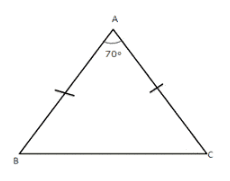$\Rightarrow \angle B=\angle C$ [angles opposites to equal sides]

But $\angle A+\angle B+\angle C=180^{\circ}$

$\Rightarrow 70^{\circ}+\angle B+\angle B=180^{\circ}$

$\Rightarrow 70^{\circ}+2\angle B=180^{\circ}$

$\Rightarrow 2\angle B=180^{\circ}-70^{\circ}$

$\Rightarrow 2\angle B=110^{\circ}$

$\Rightarrow \angle B=\frac{110^{\circ}}{2}$

$\Rightarrow \angle B=55^{\circ}$

$\Rightarrow \angle B=\angle C=55^{\circ}$

Question 2:

The vertical angle of an isosceles triangle is 100o. Find its base angles.

Solution: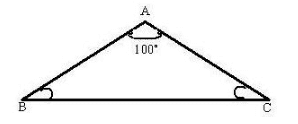Consider the isosceles triangle $\Delta ABC$.

Since the vertical angle of ABC is 100o, we have, $\angle A$ = 100o

By angle sum property of a triangle, we have,

$\angle A+\angle B+\angle C=180^{\circ}$

$\Rightarrow 100^{\circ}+\angle B+\angle C=180^{\circ}$

$\Rightarrow 100^{\circ}+2\angle B=180^{\circ}$ [since in an isosceles triangle base angles are equal, $\angle B=\angle C$]

$\Rightarrow 2\angle B=180^{\circ}-100^{\circ}=80^{\circ}$

$\Rightarrow \angle B=\frac{80^{\circ}}{2}$

$\Rightarrow \angle B=40^{\circ}$

$\Rightarrow \angle B=\angle C=40^{\circ}$

Question 3:

In a $\Delta$ABC, if AB = AC and $\angle$B = 65o, find $\angle$C and $\angle$A.

Solution: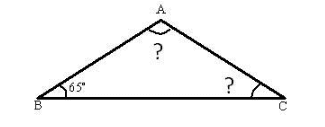In $\Delta ABC$, if AB = AC

$\Rightarrow \Delta ABC$ is an isosceles triangle

$\Rightarrow$ Base angles are equals

$\Rightarrow \angle B=\angle C$

$\Rightarrow \angle C=65^{\circ}$ [Since $\angle B=65^{\circ}$]

Also by angle sum property, we have

$\angle A+\angle B+\angle C=180^{\circ}$

$\Rightarrow \angle A+65^{\circ}+65^{\circ}=180^{\circ}$ [$\angle B=\angle C=65^{\circ}$]

$\Rightarrow \angle A=180^{\circ}-130^{\circ}=50^{\circ}$

Question 4:

In an isosceles triangle, if the vertex angle is twice the sum of the base angles, calculate the angles of the triangle.

Solution: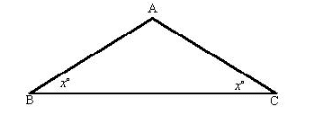Let ABC be an isosceles triangle in which AB = AC.

Then we have $\angle B=\angle C$

Let $\angle B=\angle C=x$

Then vertex angle A = 2(x + x) = 4x

Now, x + x + 4x = 180o

$\Rightarrow 6x=180^{\circ}$

$\Rightarrow x=\frac{180^{\circ}}{6}=30^{\circ}$

Therefore, Vertex $\angle A=4\times 30=120^{\circ}$

And, $\angle B=\angle C=30^{\circ}$

Question 5:

What is the measure of each of the equal angles of a right-angled isosceles triangle?

Solution: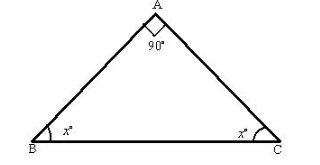In a right angle isosceles triangle, the vertex angle is $\angle A=90^{\circ}$ and the other two base angles are equal.

Let xo be the base angle and we have, $\angle B=\angle C=x$.

By angle sum property of a triangle, we have

$\angle A+\angle B+\angle C=180^{\circ}$

$\Rightarrow 90^{\circ}+x+x=180^{\circ}$

$\Rightarrow 90^{\circ}+2x=180^{\circ}$

$\Rightarrow 2x=180^{\circ}-90^{\circ}=90^{\circ}$

$\Rightarrow x=\frac{90^{\circ}}{2}=45^{\circ}$

Thus, we have $\angle B=\angle C=45^{\circ}$

Question 6:

If the base of an isosceles triangle is produced on both sides, prove that the exterior angles so formed are equal to each other.

Solution:

Given: ABC is an isosceles triangle in which AB = AC and BC has produced both ways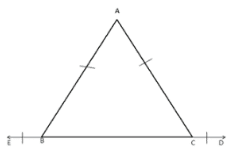To Prove: $\angle$EBA = $\angle$DCA

Proof: In $\Delta$ABC we have,

AB = AC

$\Rightarrow$ $\angle$B = $\angle$C

Now exterior $\angle$EBA = $\angle$A + $\angle$C = $\angle$A + $\angle$B [$\angle$B = $\angle$C]

and exterior $\angle$DCA = $\angle$A + $\angle$B

$\Rightarrow$ Exterior $\angle$EBA = Exterior $\angle$DCA.

Question 7:

Find the measure of each exterior angle of an equilateral triangle.

Solution: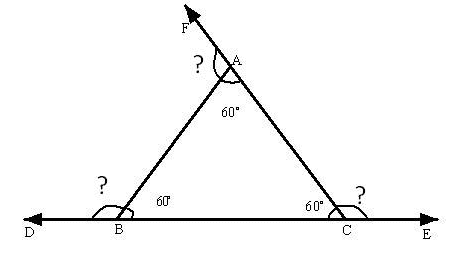Let $\Delta ABC$ be an equilateral triangle.

Since it is an equilateral triangle, all the angle is 60o

The exterior angle of $\angle A$ = $\angle BAF$

The exterior angle of $\angle B$ = $\angle ABD$

The exterior angle of $\angle C$ = $\angle ACE$

We can observe that angles $\angle A$ and $\angle BAF$, $\angle B$ and $\angle ABD$, $\angle C$ and $\angle ACE$ form linear pairs.

Therefore, we have

$\angle A+\angle BAF=180^{\circ}$

$\Rightarrow 60^{\circ}+\angle BAF=180^{\circ}$

$\Rightarrow \angle BAF=180^{\circ}-60^{\circ}=120^{\circ}$

Similarly, we have

$\angle B+\angle ABD=180^{\circ}$

$\Rightarrow 60^{\circ}+\angle ABD=180^{\circ}$

$\Rightarrow \angle ABD=180^{\circ}-60^{\circ}=120^{\circ}$

Also, we have

$\angle C+\angle ACE=180^{\circ}$

$\Rightarrow 60^{\circ}+\angle ACE=180^{\circ}$

$\Rightarrow \angle ACE=180^{\circ}-60^{\circ}=120^{\circ}$

Thus, we have, $\angle BAF=120^{\circ}$, $\angle ABD=120^{\circ}$, $\angle ACE=120^{\circ}$

So, the measure of each exterior angle of an equilateral triangle is 120o.

Question 8:

In the given figure, O is the mid-point of each of the line segments AB and CD. Prove that AC = BD and AC || BD.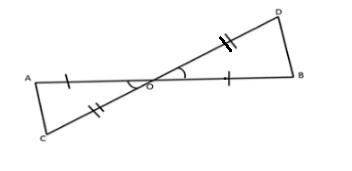Solution:

Given: Two lines AB and CD intersect at O and O is the midpoint of AB and CD.

$\Rightarrow$ AO = OB and CO = OD

To prove: AC = BD and AC || BD

Proof:

In $\Delta$AOC and $\Delta$BOD, we have,

AO = OB [Given: O is the midpoint of AB]

$\angle$AOC = $\angle$BOD [Vertically opposite angles]

CO = OD [Given: O is the midpoint of CD]

So, by Side-Angle-Side congruence triangle are equal.

Therefore, we have, AC = BD.

Similarly, by c.p.c.t, we have, $\angle$ACO = $\angle$BDO and $\angle$CAO = $\angle$DDBO

This implies that alternate angles formed by AC and BD with transversal CD are equal. This means that, AC || BD. Thus, AC = BD and AC || BD.

### RS Aggarwal Class 9 Solutions Chapter 5 – Congruence Of Triangles and Inequalities In Triangle

The solutions of Class prove to be highly effective in enabling students to prepare for the difficult topics in a proper way. All these solutions play a vital role in helping students solve difficult questions in a quick time. Using our solutions for Class 9 Maths, you can clear your doubts well and attempt good number of questions during the exam. Students can rely on RS Aggarwal Maths Solution to score good marks in the exam. The solutions are derived in a step by step approach for better and effortless understanding.